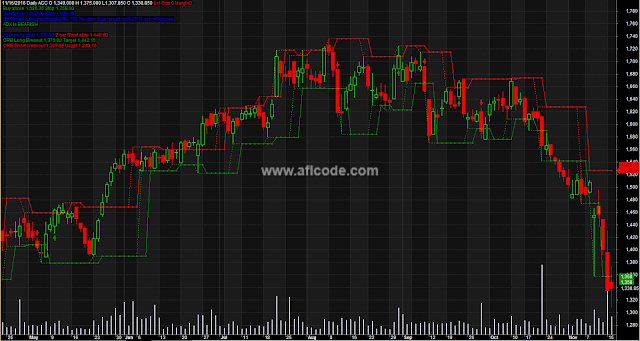### Composite Volume Support Resistance SystemComposite Volume Support Resistance System

```//www.aflcode.com
SetChartOptions(1,chartShowDates);

_SECTION_BEGIN("POS SIZE");
cap = Param("Capital", 0,100000, 5000000, 50000 );
risk = Param("Max. Risk",0.0025, 0.005, 0.02, 0.0025 );
/*The following builds Fractal Up*/

var1=ValueWhen(

(Ref(H,-2) > Ref(H, -4)) AND

(Ref(H,-2) > Ref(H, -3)) AND

(Ref(H,-2) > Ref(H, -1)) AND

(Ref(H,-2) > H), Ref(H,-2),1);

FractalUp=HighestSince(var1>0,var1,1);
Plot(FractalUp,"Res",colorRed,8);

/*The following builds Fractal Down*/

var2=

(Ref(L,-2) <= Ref(L, -1)) AND

(Ref(L,-2) <= Ref(L, 0)) AND

(Ref(L,-2) <= Ref(L, -3)) AND

(Ref(L,-2) <= Ref(L, -4));

FractalDown=ValueWhen( var2,Ref(L,-2),1);
Plot(FractalDown,"Supp",colorGreen,8);
a=LLV(L,3);
a1=HHV(H,3);
TimeFrameRestore();

Plot(C,"",colorBlack,styleCandle);

_SECTION_BEGIN("Volume"); Plot( Volume, "Volume", ParamColor("Color", colorLavender ), styleNoTitle | ParamStyle( "Style", styleHistogram | styleOwnScale | styleThick | styleNoLabel, maskHistogram ), 5 );

dn = DateNum();
u = ValueWhen( tn<105500, HighestSince(dn!=Ref(dn,-1), H ));
w = ValueWhen( tn<105500, LowestSince(dn!=Ref(dn,-1), L ));
Longtgt=u+(u-w);
Shrttgt=w-(u-w);
Cond13=PDI()>MDI();

Title = Date()+" "+ Interval(format=2)+" "+Name()+" "+"O "+WriteVal(O)+" "+"H "+WriteVal(H)+" L"+WriteVal(L)+" C "+WriteVal(C)+EncodeColor(colorRed)+" Lot Size "+WriteVal(RoundLotSize,1.0)+" Margin"+WriteVal(MarginDeposit,1.0)+"\n"
+EncodeColor(colorGreen)+" Buy above "+WriteVal(FractalUp,1.2)+" Stop "+WriteVal(FractalDown,1.2)+ "\n"+EncodeColor(colorBlue)+" Sell below "+WriteVal(FractalDown,1.2)+ " Stop "+WriteVal(FractalUp,1.2)+
"\n"+" Trade risk based on fractals Rs "+ WriteVal((FractalUp-FractalDown),1.0)+ " Position Size based on " + WriteVal(risk*100,1.2)+ " % risk = " + WriteVal((cap*risk)/(FractalUp-FractalDown),1.0)+ "shares"+
"\n"+ EncodeColor(colorGreen)+" ADX is "+ WriteIf(Cond13,"BULLISH","BEARISH ")+
"\n"+EncodeColor(colorBlue)+
"\n"+"2 bar Long stop " +WriteVal(a,1.2)+EncodeColor(colorRed)+" 2 bar Short stop "+WriteVal(a1,1.2)+
"\n"+EncodeColor(colorGreen)+ " ORB Long Breaout "+WriteVal(u,1.2)+" Target "+ WriteVal(Longtgt,1.2)
+"\n"+EncodeColor(colorRed)+" ORB Short breakout "+WriteVal(w,1.2)+" target "+ WriteVal(Shrttgt,1.2);

// Previous version 1.0 on 28.07.2008.
// Current Version 1.1 on 31.07.2008.

///////////////////////////////////////////////////////////
///// Major changes done from previous version 1.0 : //////

// The method of pivot definition is changed.
// Now it does not use zig() or related functions anywhere.

// Stoploss line error, that it moves in both directions after a position is taken,
// is removed. Now stoploss lines are unidirectional.

// To protect profit on long positions, SELL is triggered either when trailing buy stop
// is hit OR when previous lower pivot is cracked. Fresh long position will be initiated
// if uptrend continues after previous high pivot is cracked.

///////////////////////////////////////////////////////////

// This is simple YET powerful pivot based trading system.
// Used only for intraday. Not suitable for EOD data.
// When long, watch crack of close in green line ONLY. Ignore red line.
// When short, watch crack of close in red line ONLY. Ignore green line.
// You will have to tweak the parameters as per your trading style.

// Warning :  I have tried to develop this method from Saint's Pivot Based System (www.traderji.com)
//   However, it is very well possible that its performance is not as per actual system
//   suggested by Saint due to programming errors and inadequate knowledge level at my end.
// Warning :  Traders are advised to trade on their own, knowing very well that it is their money.

// User defined parameters.

GraphXSpace = 10; //defines how much extra space should be added above and below graph line (in percent).

dist = 0.25*ATR(10);
Capital=Param("Total capital",100000,10000,1000000,1000);
drawdown=Param("Max. loss per trade as % of Capital", 1.0,0.5,25.0,0.1);
room=Param("Room for S/L as % of Pivot value",0,0.001,0.02,0.001);

// Now calculate pivots. Method courtesy : Kenneth (www.traderji.com)

PH= ValueWhen(
(Ref(H,-2) > Ref(H, -4)) AND
(Ref(H,-2) > Ref(H, -3)) AND
(Ref(H,-2) > Ref(H, -1)) AND
(Ref(H,-2) > H), Ref(H,-2));

PL= ValueWhen(
(Ref(L,-2) <= Ref(L, -4)) AND
(Ref(L,-2) <= Ref(L, -3)) AND
(Ref(L,-2) <= Ref(L, -1)) AND
(Ref(L,-2) <= L), Ref(L,-2));

// filter lines

phfilter=PH+(room*PH);
plfilter=PL-(room*PL);

//Uncomment following code if you wish to see the pivot lines.
Plot(Ref(PH,2), "UpPivot", ParamColor("UpPivot Color",colorRed), styleDashed);
Plot(Ref(PL,2), "DownPivot",ParamColor("DownPivot Color",colorGreen), styleDashed);

//Plot(Ref(Phfilter,2), "Upfilter", ParamColor("upfilter Color",colorRed), styleLine);
//Plot(Ref(Plfilter,2), "Downfilter",ParamColor("dnfilter Color",colorGreen), styleLine);

//Plot(Ref(UpFractal,2), "Up Fractal", ParamColor("Up Fractal Color",colorRed),8);
//Plot(Ref(DownFractal,2), "Down Fractal",ParamColor("Down Fractal Color",colorGreen),8);

/*for (a=4;a<BarCount;a++)
{
if ((H[a-2] >= H[a-4]) AND
(H[a-2] >= H[a-3]) AND
(H[a-2] >= H[a-1]) AND
(H[a-2] >= H[a]))
PlotText("PH", a-2, H[a-2], colorGreen);

if ((L[a-2] <= L[a-4]) AND
(L[a-2] <= L[a-3]) AND
(L[a-2] <= L[a-1]) AND
(L[a-2] <= L[a]))
PlotText("PL", a-2, L[a-2]-dist[a-2], colorRed);
}*/

//Condition for buy : Enter when Close crosses latest pivot high.

//Condition for sell : Exit when previous pivot low is cracked.
Sell = C< (PL-(room*PL));

//Short = Sell;